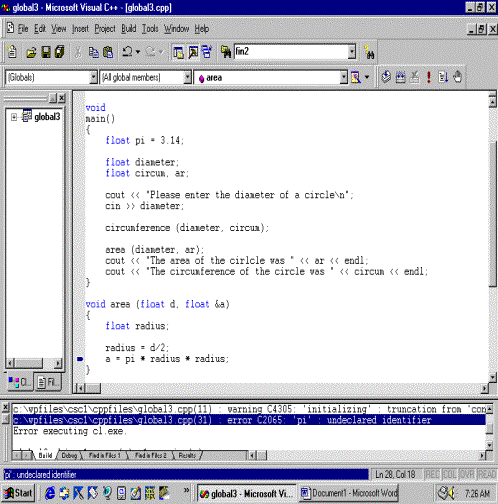Math Library functions

#include <cmath>

To use:

1.     need to know name of function

2.     what the function does

3.     type of the data required by function

4.     data type of result

Want to find the square root of a number.  sqrt(x) where x is any numeric data type.

f(x)   here x represents data to function.

functionname( function argument)

Arguments are the means by which the function gets information from and gives information back to the calling function.

int num;

num = sqrt(4);

num = sqrt(4.2);

Accepts both int and float.  Data type of result is same as the argument.

common functions:

found in cmath  #include <cmath>

abs(x)    returns the absolute value of an integer

fabs(x) returns the absolute value of a float or double.

ceil(x) returns the smallest integer that is not less than a

floor(x) returns the smallest integer that is not greater than a

pow(x, y) returns a raised to b power

sqrt(x) returns square root of a

sin(x) returns sine of a

cos(x)cosine

tan(x) tangent

found in cctype library

tolower(x) -  returns lower case value of a if a is upper case otherwise returns x.

toupper(x) -  returns upper case value of a if a is lower case otherwise returns x

found in cstdlib

rand( );  srand( x );

psuedorandom number generator - will eventually start repeating itself.

rand will generate a series of seemingly random numbers depending on the number it starts with.  If rand starts with the same number each time, it will generate the same sequence of numbers.

srand(x) is a function in which x is the seed or starting number for the random number generator.  If srand is not used, rand assumes a seed of 1.

srand allows you to start with different starting points, allowing the random number generator to seem more random.   srand must only be used once or the random number generator may not be random.

Evaluating multiple functions

num1 = sqrt( pow( abs(num2), num1)); //evaluated according to rules for parenthesized expressions.

User - defined functions

Problem:  You wish to make a nice card for your mother for the holidays.  You decide to show off your new found computer skills by printing it on your computer.  You write a C++ program that will print the word MOM as such.

*       *

* *   * *

*   *   *

*      *

*       *

* * *

*       *

*       *

*       *

* * *

*       *

* *   * *

*   *   *

*      *

*       *

Program to print MOM in asterisks

#include <iostream>

using namespace std;

int

main()

{

//Print an M

cout << "*   *\n";

cout << "** **\n";

cout << "* * *\n";

cout << "*   *\n";

cout << "*   *\n\n";

//Print an O

cout << " *** \n";

cout << "*   *\n";

cout << "*   *\n";

cout << "*   *\n";

cout << " *** \n\n";

//Print an M

cout << "*   *\n";

cout << "** **\n";

cout << "* * *\n";

cout << "*   *\n";

cout << "*   *\n\n";

return 0;

}

//Program to print MOMMY in asterisks

#include <iostream>

using namespace std;

void print_M();

void print_O();

void print_Y();

int

main()

{

//Print the word MOMMY in asterisks

print_M();

print_O();

print_M();

print_M();

print_Y();

return 0;

}

void

print_M()

{

//Print an M

cout << "*   *\n";

cout << "** **\n";

cout << "* * *\n";

cout << "*   *\n";

cout << "*   *\n\n";

}

void

print_O()

{

//Print an O

cout << " *** \n";

cout << "*   *\n";

cout << "*   *\n";

cout << "*   *\n";

cout << " *** \n\n";

}

void

print_Y()

{

//Print a Y

cout << "*   *\n";

cout << " * * \n";

cout << "  *  \n";

cout << "  *  \n";

cout << "  *  \n\n";

}

A C function groups program statements that logically perform a specific task.

The syntax of  a simple function.

void

functionname (void)

{

stmt1;

stmt2;

.

.

stmt n:

}

Invoking or calling a function

When we invoke or call a function we mean to execute statements from within that function.

Flow of control - The sequence of steps followed during a program's execution.  The flow of control of the same program may be different depending on variable values, decisions, function calls, etc.

When we call or invoke a function, the flow of control changes from the calling function to the invoked function.

The function prototype is placed before the main function.  A function which calls another function (i.e. the main function calling another function) must know about the called function.  Therefore the called function must be declared before a calling function.

The function code is placed after the closing bracket of the main function.

1.     Allows for modular programming.  Each function can solve a specific sub problem.

a.      easier implementation of algorithm

b.     easier to debug.  A piece of code that is working will continue to work.

c.     A change in the sub problem necessitates changing the function and not the whole program.

2.     If statements are repeatedly executed, we need write the statements once and then repeatedly execute them with a call statement.

3.     Saves memory

Functions That Communicate.

Communicating to a function.

void functionname ( formal parameter list);

int

main( )

{

.

.

functionname( actual parameter list)

.

return 0;

}

void

functionname (   formal parameter list )

{

}

Problem:  Write a C++ program that has a function called add5.  The main function prompts the user for a number and calls function add5.  add5 increments the number by 5 and prints the result.

#include <iostream>

using namespace std;

void

main( )

{

int num;

cout << "Please enter a value: ";

cin >> num;

}

//Input: integer  Output: the integer incremented by 5

void

{

n = n + 5;

cout << "Five added to " << n << " is " << n2 << endl;

}

add5 is a function with one input value and no output value.

What happens in memory?  When function add5 is called, memory is allocated for formal parameter n.  A copy of the value in num is placed in this memory location.  When add5 finishes, the memory allocated to n is then deallocated.  n is called a value parameter.

Problem: Write a C++ program that uses a function small to find the smaller of 3 numbers.  small prints the results.

#include <iostream>

using namespace std;

void small (int, int, int);

void

main()

{

int num1, num2, num3;

cout << "Please three values: ";

cin >> num1 >>  num2 >> num3;

small (num1, num2, num3);

}

void

small (int n1, int n2, int n3)

{

int smallest;

if (n1 > n2) smallest = n2;

else smallest = n1;

if (smallest > n3) smallest = n3;

cout << "The smallest number is: " << smallest << endl;

}

We can declare variables from within a function.  These are known as local variables.  When the function finishes execution, memory for these variables is deallocated.

Note:

1.        The number of the actual arguments must match the number of formal parameters

2.        There is a 1:1 correspondence between the actual argument and formal parameters.

3.        When assigning a value from an actual parameter to a formal parameter the data types must be such that there is no loss of data between the two.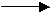i.e.  int       float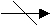float         int

4.        Void functions do not use the return statement to communicate results.

Problem:  We wish to write a C++ program that uses a function to calculate any triangular number.  A triangular number is the sum of all integers from 1 up to and including that number.  The results are printed from the main function.

 Number Triangular Number 1 1 2 3 3 6 4 10 5 15

We need to communicate the function results back to the main function.

int

main

{

.

.

return 0;

}

We can use a return statement to return a single value back to the calling function.  The data type of the function is the data type of the returned value.
#include <iostream>

using namespace std;

int calc_triangular (int n);

void

main()

{

int num, triangular;

cout << "Please enter a number: ";

cin >> num;

triangular = calc_triangular(num);

cout << "The triangular value for the number "<< num

<< " is " << triangular << endl;

}

//This function will calculate a triangular number

//Inputs: integer   Outputs: the triangular number

int

calc_triangular (int n)

{

int i, trinum;

trinum = 0;

for (i = 1; i <= n; ++i)

trinum += i;

return (trinum);

}

What happens in memory?

main data area                                              calc_traiangular data area

 trinum

 4

 i

 n(created when function is called)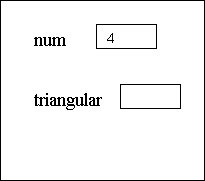num and n are two different unrelated areas in memory.  Any changes to n DO NOT affect num.  n is called a value parameter.  When using formal value parameters, a copy of the actual parameter's value is passed to the value parameter.  Changes in the function to the value parameter do not affect the variable in the calling function.

Problem:  The greatest common denominator of two numbers is the largest number that can evenly divide two numbers.  Write a C++ program that uses a function to calculate the gcd of two numbers.  The results are printed from the main function.

Euclidean algorithm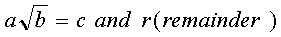gcd(a,b) = gcd(b,r)

gcd(num1, num2) = gcd( num2, num1 % num2)

while num 2 does not equal 0

1.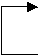rem = num1 % num2

2.
 while num2 != 0

num1 = num2

3.     num2 = rem

#include <iostream>

using namespace std;

int gcd (int n1, int n2);

void

main()

{

int num1, num2;

cout << "Please enter two numbers: ";

cin >> num1 >>num2;

cout << "The gcd of " << num1 << " and " << num2 << " is "

<< gcd(num1, num2) << endl;

}

int

gcd (int n1, int n2)

{

int rem;

while (n2 != 0)

{

rem = n1 % n2;

n1 = n2;

n2 = rem;

}

return (n1);

}

What happens when the formal and actual parameter lists have the same variable names?

#include <iostream>

using namespace std;

int gcd (int, int);

void

main()

{

int num1, num2;

cout << "Please enter two numbers: ";

cin >> num1 >>num2;

cout << "The gcd of " << num1 << " and " << num2 << " is "

<< gcd(num1, num2) << endl;

}

int

gcd (int num1, int num2)

{

int rem;

while (num2 != 0)

{

rem = num1 % num2;

num1 = num2;

num2 = rem;

}

return (num1);

}

main memory data area                                 gcd memory data area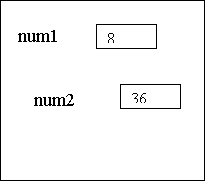How can we return more than one result from a function?

Value parameter - copy of value of argument is passed to the parameter.  Changes in the function to the parameter do not affect the variable in the calling function.

Reference parameter - associates another name with the argument variable.  Changes to the reference parameter affect the associated variable in the calling function.

Syntax:

function call

assignnew (a,b);

void assignnew (int &d, int &e);

& indicates reference parameter

#include <iostream>

using namespace std;

void assignnew  (char &x, char &y);

int

main()

{

char let1, let2;

let1 = 'k';

let2 = 'p';

cout << "let1 = " << let1 << endl;

cout << "let2 = " << let2 << endl;

assignnew (let1, let2);

cout << "let1 = " << let1 << endl;

cout << "let2 = " << let2 << endl;

return 0;

}

void assignnew (char &alpha2, char &alpha1)

{

cout << "alpha2 = " << alpha2 << endl;

cout << "alpha1 = " << alpha1 << endl;

alpha2 = 'w';

alpha1 = 'r';

cout << "alpha2 = " << alpha2 << endl;

cout << "alpha1 = " << alpha1 << endl;

}

let1 = k

let2 = p

alpha2 = k

alpha1 = p

alpha2 = w

alpha1 = r

let1 = w

let2 = r

Press any key to continue

Global Variables

#include <iostream>

using namespace std;

void circumference (float d, float &c);

void area (float d, float &c);

float pi = 3.14;

void

main()

{

float diameter;

float circum, ar;

cout << "Please enter the diameter of a circle\n";

cin >> diameter;

circumference (diameter, circum);

area (diameter, ar);

cout << "The area of the cirlcle was " << ar << endl;

cout << "The circumference of the circle was " << circum << endl;

}

void area (float d, float &a)

{

}

void circumference (float d, float &c)

{

c = 2 * pi * radius;

}

Please enter the diameter of a circle

10

The area of the cirlcle was 78.5

The circumference of the circle was 31.4

Press any key to continue

The use of global variables has certain side effects.  A function using a global variable is not independent and can't be used in other programs easily.  Use of global variables can make a program difficult to debug.  Therefore, the use global variables is discouraged when you can pass parameters.

The scope of a variable consists of the section of code that can reference that variable.  A global variable has scope over all sections of code placed after the variable definition.  When a variable of the same name as a global variable,  is declared locally, the local definition takes precedence over the global definition.

#include <iostream>

using namespace std;

void circumference (float d, float &c);

void area (float d, float &c);

float pi = 3.14;

void

main()

{

float diameter;

float circum, ar;

cout << "Please enter the diameter of a circle\n";

cin >> diameter;

circumference (diameter, circum);

area (diameter, ar);

cout << "The area of the circle was " << ar << endl;

cout << "The circumference of the circle was " << circum << endl;

}

void area (float d, float &a)

{

}

void circumference (float d, float &c)

{

float pi;

pi = float(22)/7;

c = 2 * pi * radius;

}

Please enter the diameter of a circle

10

The area of the circle was 78.5

The circumference of the circle was 31.4286

Press any key to continue

Variables declared in a function are only have scope within that function.

#include <iostream>

using namespace std;

void circumference (float d, float &c);

void area (float d, float &c);

void

main()

{

float pi = 3.14;

float diameter;

float circum, ar;

cout << "Please enter the diameter of a circle\n";

cin >> diameter;

circumference (diameter, circum);

area (diameter, ar);

cout << "The area of the circle was " << ar << endl;

cout << "The circumference of the circle was " << circum << endl;

}

void area (float d, float &a)

{

}

void circumference (float d, float &c)

{

c = 2 * pi * radius;

}